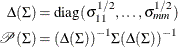# The COPULA Procedure

### Normal Copula

Subsections:

Let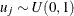for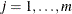, where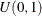represents the uniform distribution on the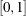interval. Let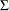be the correlation matrix with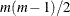parameters satisfying the positive semidefiniteness constraint. The normal copula can be written as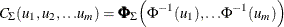where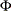is the distribution function of a standard normal random variable and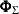is the m-variate standard normal distribution with mean vector 0 and covariance matrix. That is, the distributionis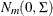.

#### Simulation

For the normal copula, the input of the simulation is the correlation matrix. The normal copula can be simulated by the following steps in which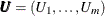denotes one random draw from the copula:

1. Generate a multivariate normal vector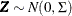whereis an m-dimensional correlation matrix.

2. Transform the vector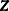into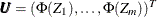, whereis the distribution function of univariate standard normal.

The first step can be achieved by Cholesky decomposition of the correlation matrix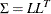where
is a lower triangular matrix with positive elements on the diagonal. If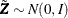, then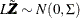.

#### Fitting

To fit a normal copula is to estimate the covariance matrixfrom an input sample data set. Given a random sample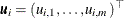where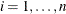, the log-likelihood function is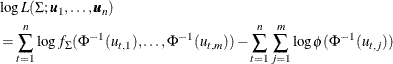Here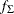is the joint density of the multivariate normal with mean zero and variance, andis the univariate density of the standard normal distribution. Note that the second term is not related to the parametersand, therefore, can be ignored during the optimization. The restriction thatis a correlation matrix is very inconvenient, and it is common practice to circumvent this problem by first assuming thathas the covariance form. Therefore,can be estimated by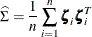where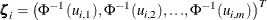This estimate is consistent with the form of a covariance matrix but not necessarily with the form of a correlation matrix. The approximation to the original MLE problem can be obtained using the normalizing operator defined as follows: